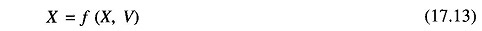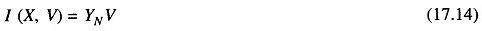## Voltage Stability Analysis:

The voltage stability analysis for a given system state involves examining following two aspects.

• Proximity to voltage instability: Distance to instability may be measured in terms of physical quantities, such as load level, real power flow through a critical interface, and reactive power reserve. Possible contingencies, such as a line outage, loss of a generating unit or a reactive power source must be given due consideration.
• Mechanism of voltage instability: How and why does voltage instability take place? What are the main factors leading to instability? What are the voltage-weak areas? What are the most effective ways to improve voltage stability?

The static analysis techniques permit examination of a wide range of system conditions and can describe the nature of the problem and give the main contributing factors. Dynamic analysis is useful for detailed study of specific voltage collapse situations, coordination of protection and controls, and testing of remedial measures. Dynamic simulations further tell us whether and how the steady-state  equilibrium point will be reached.

### Modelling Requirements of Various Power System Components Loads:

Load modelling is very critical in voltage stability analysis. Detailed sub transmission system representation in a voltage-weak area may be required. This may include transformer ULTC action, reactive power compensation, and voltage regulators.

It is essential to consider the voltage and frequency dependence of loads. Induction motors should also be modelled.

### Generators and their excitation controls:

It is necessary to consider the droop characteristics of the AVR, load compensation, SVSs (static var system), AGC, protection and controls should also be modelled appropriately.

### Dynamic Analysis:

The general structure of the system model for voltage stability analysis is similar to that for transient stability analysis. Overall system equations may be expressed asand a set of algebraic equationswith a set of known initial conditions (X0, V0).

where

• X = system state vector
• V = bus voltage vector
• I = current injection vector
• YN = network node admittance matrix.

Equations (17.13) and (17.14) can be solved in time domain by employing any of the numerical integration methods and power flow analysis methods. The study period is of the order of several minutes. As the special models representing the “slow system dynamics” leading to voltage collapse have been included, the stiffness of the system differential equations is considerably higher than that of transient stability models. Stiffness is also called synchronizing coefficient.

### Static Analysis:

The static approach captures snapshots of system conditions at various time frames along the time-domain trajectory. At each of these time frames, X in Eq. (17.13) is assumed to be zero, and the state variables take on values appropriate to the specific time frame. Thus, the overall system equations reduce to purely algebraic equations allowing the use of static analysis techniques.

In static analysis voltage stability is determined by computing VP and VQ curves at selected load buses. Special techniques using static analysis have been reported in literature. Methods based on VQ sensitivity such as eigenvalue (or modal) analysis have been devised. These methods give stability-related information from a system-wide perspective and also identify areas of potential problems.

### Proximity to Instability:

Proximity to small-disturbance voltage instability is determined by increasing load-generation in steps until the system becomes unstable or the load flow fails to converge discuss special techniques for determining the point of voltage collapse and proximity to voltage instability.

### The Continuation Power-flow Analysis:

The Jacobian matrix becomes singular at the voltage stability limit. As a result, conventional load-flow algorithms may have convergence problems at operating conditions near the stability limit. The continuation power-flow analysis overcomes this problem by reformulating the load-flow equations so that they remain well-conditioned at all possible loading conditions. This allows the solution of load-flow problem for both upper and lower portions of the P-V curve.

The continuation method of power-flow analysis is robust and flexible and suited for solving load flow problems with convergence difficulties. However, the method is very slow and time-consuming. Hence the better approach is to use combination of conventional load flow method (NR/FDLF) and continua­tion method. Starting from the base case, LF is solved using a conventional method to compute power flow solutions for successively increasing load levels until a solution cannot be obtained. Hereafter, the continuation method is resorted to obtain the load-flow solutions. Normally, the continuation method is required only if solutions are required exactly at and past the critical point.

### Voltage Stability with HVDC Links:

High voltage direct current (HVDC) links are used for extremely long distance transmission and for asynchronous interconnections. An HVDC link can be either a back-to-back rectifier/inverter link or can include long distance dc transmission. Multi-terminal HVDC links are also feasible.

The technology has come to such a level that HVDC terminals can be connected even at voltage-weak points in power systems. HVDC links may present unfavorable “load” characteristics to the power system as HVDC converter consumes reactive power equal to 50-60% of the dc power.

HVDC-related voltage control (voltage stability and fundamental frequency temporary over voltages) may be studied using a transient stability program. Transient stability is often interrelated with voltage stability considers this problem in greater detail.

Scroll to Top